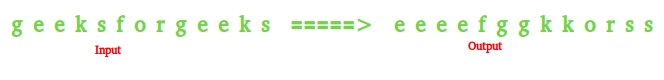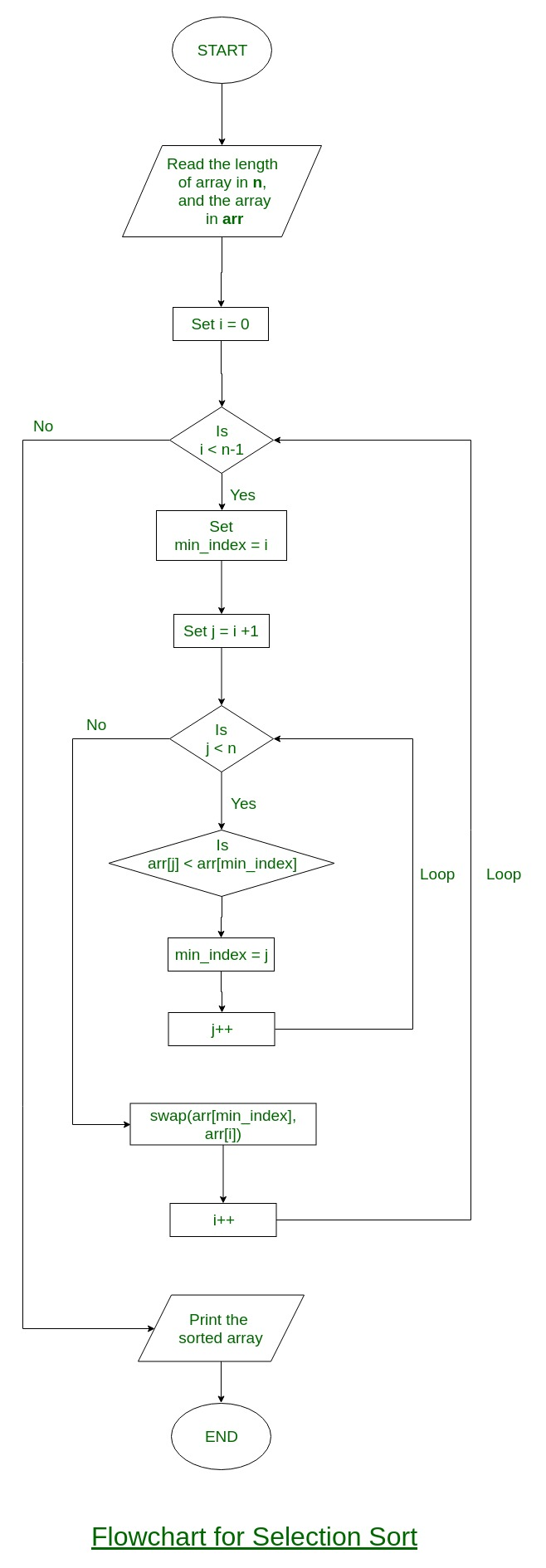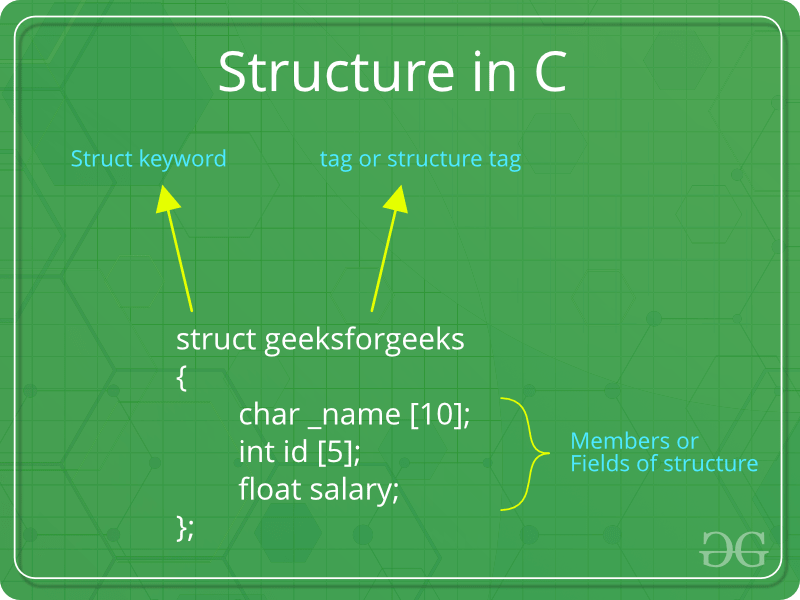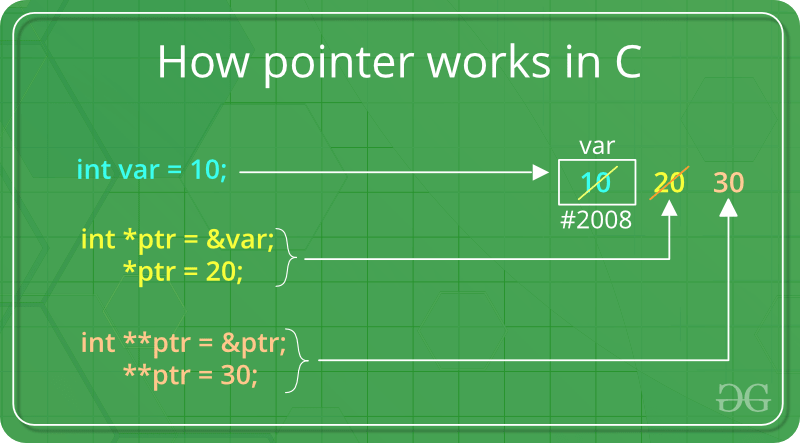# AKTU 1st Year Sem 1 Solved Paper 2016-17 | COMP. SYSTEM & C PROGRAMMING | Sec C

• Last Updated : 30 Jan, 2019

# B.Tech.(SEM-II) THEORY EXAMINATION 2016-17COMPUTER SYSTEM & PROGRAMMING IN C

Time: 3hrs
Total Marks: 100

Note:-

• There are three sections. Section A carries 20 marks, Section B carries 30 marks and Section C carries 50 marks.
• Attempt all questions. Marks are indicated against each question.
• Assume suitable data wherever necessary.

# Section – C

Attempt any two questions from this section: (2*15 = 30)

3. a) What do you mean by sorting. Write a program in C to sort the given n positive integers. Also give the flowchart for the same.

A Sorting Algorithm is used to rearrange a given array or list elements according to a comparison operator on the elements. The comparison operator is used to decide the new order of element in the respective data structure.

For example: The below list of characters is sorted in increasing order of their ASCII values. That is, the character with lesser ASCII value will be placed first than the character with higher ASCII value.Flowchart of the Selection Sort:Program in C to sort the elements of a given array of N positive integers:

 `// C program to sort the elements``// of a given array of N positive integers`` ` `#include `` ` `void` `swap(``int``* xp, ``int``* yp)``{``    ``int` `temp = *xp;``    ``*xp = *yp;``    ``*yp = temp;``}`` ` `void` `selectionSort(``int` `arr[], ``int` `n)``{``    ``int` `i, j, min_idx;`` ` `    ``// One by one move boundary of unsorted subarray``    ``for` `(i = 0; i < n - 1; i++) {``        ``// Find the minimum element in unsorted array``        ``min_idx = i;``        ``for` `(j = i + 1; j < n; j++)``            ``if` `(arr[j] < arr[min_idx])``                ``min_idx = j;`` ` `        ``// Swap the found minimum element with the first element``        ``swap(&arr[min_idx], &arr[i]);``    ``}``}`` ` `/* Function to print an array */``void` `printArray(``int` `arr[], ``int` `size)``{``    ``int` `i;``    ``for` `(i = 0; i < size; i++)``        ``printf``(``"%d "``, arr[i]);``    ``printf``(``"\n"``);``}`` ` `// Driver program to test above functions``int` `main()``{``    ``int` `arr[] = { 64, 25, 12, 22, 11 };``    ``int` `n = ``sizeof``(arr) / ``sizeof``(arr);``    ``selectionSort(arr, n);``    ``printf``(``"Sorted array: \n"``);``    ``printArray(arr, n);``    ``return` `0;``}`
Output:
```Sorted array:
11 12 22 25 64
```

3. b) Write a program to check whether a given number is Armstrong or not. Like 153 = 13 + 53 + 33.

 `// C program to find Armstrong number`` ` `#include `` ` `/* Function to calculate x raised to the power y */``int` `power(``int` `x, unsigned ``int` `y)``{``    ``if` `(y == 0)``        ``return` `1;``    ``if` `(y % 2 == 0)``        ``return` `power(x, y / 2) * power(x, y / 2);``    ``return` `x * power(x, y / 2) * power(x, y / 2);``}`` ` `/* Function to calculate order of the number */``int` `order(``int` `x)``{``    ``int` `n = 0;``    ``while` `(x) {``        ``n++;``        ``x = x / 10;``    ``}``    ``return` `n;``}`` ` `// Function to check whether the given number is``// Armstrong number or not``int` `isArmstrong(``int` `x)``{``    ``// Calling order function``    ``int` `n = order(x);``    ``int` `temp = x, sum = 0;``    ``while` `(temp) {``        ``int` `r = temp % 10;``        ``sum += power(r, n);``        ``temp = temp / 10;``    ``}`` ` `    ``// If satisfies Armstrong condition``    ``if` `(sum == x)``        ``return` `1;``    ``else``        ``return` `0;``}`` ` `// Driver Program``int` `main()``{``    ``int` `x = 153;``    ``if` `(isArmstrong(x) == 1)``        ``printf``(``"True\n"``);``    ``else``        ``printf``(``"False\n"``);`` ` `    ``x = 1253;``    ``if` `(isArmstrong(x) == 1)``        ``printf``(``"True\n"``);``    ``else``        ``printf``(``"False\n"``);`` ` `    ``return` `0;``}`
Output:
```True
False
```

4. a) Define a structure? Write a program in C to create a database of fifty students to store personal details such as roll no., name and marks. Print all the details of students whose name is entered by the user.

A structure is a user-defined data type in C/C++. A structure creates a data type that can be used to group items of possibly different types into a single type.How to create a structure?
‘struct’ keyword is used to create a structure. Following is an example.

 `struct` `address {``    ``char` `name;``    ``char` `street;``    ``char` `city;``    ``char` `state;``    ``int` `pin;``};`

How to declare structure variables?
A structure variable can either be declared with structure declaration or as a separate declaration like basic types.

 `// A variable declaration with structure declaration.``struct` `Point {``    ``int` `x, y;``} p1; ``// The variable p1 is declared with 'Point'`` ` `// A variable declaration like basic data types``struct` `Point {``    ``int` `x, y;``};`` ` `int` `main()``{``    ``struct` `Point p1; ``// The variable p1 is declared like a normal variable``}`

Note: In C++, the struct keyword is optional before in declaration of a variable. In C, it is mandatory.

Program:

 `#include ``#include `` ` `struct` `Student {``    ``int` `roll_no;``    ``char` `name;``    ``float` `marks;``};`` ` `int` `main()``{``    ``int` `i = 0;``    ``char` `n;``    ``struct` `Student student;`` ` `    ``for` `(i = 0; i < 50; i++) {``        ``printf``(``"\nEnter details for Student %d"``, i + 1);`` ` `        ``printf``(``"\nRoll Number: "``);``        ``scanf``(``"%d"``, &student[i].roll_no);`` ` `        ``printf``(``"\nName: "``);``        ``scanf``(``"%s"``, student[i].name);`` ` `        ``printf``(``"\nMarks: "``);``        ``scanf``(``"%f"``, &student[i].marks);``    ``}`` ` `    ``printf``(``"\nEnter the name of the student whose details you need: "``);``    ``scanf``(``"%s"``, n);`` ` `    ``for` `(i = 0; i < 50; i++) {``        ``if` `(``strcmp``(n, student[i].name) == 0) {`` ` `            ``printf``(``"\nRoll Number: %d"``, student[i].roll_no);`` ` `            ``printf``(``"\nName: %s"``, student[i].name);`` ` `            ``printf``(``"\nMarks: %f"``, student[i].marks);`` ` `            ``break``;``        ``}``    ``}`` ` `    ``if` `(i == 50)``        ``printf``(``"No student found with this name"``);``}`

4. b) What do you mean by macro? Explain types of macro with its examples.

Macros: Macros are piece of code in a program which is given some name. Whenever this name is encountered by the compiler the compiler replaces the name with the actual piece of code. The ‘#define’ directive is used to define a macro. Let us now understand macro definition with the help of a program:

 `#include `` ` `// macro definition``#define LIMIT 5``int` `main()``{``    ``for` `(``int` `i = 0; i < LIMIT; i++) {``        ``std::cout << i << ``"\n"``;``    ``}`` ` `    ``return` `0;``}`
Output:
```0
1
2
3
4
```

Output:

```0
1
2
3
4
```

In the above program, when the compiler executes the word LIMIT it replaces it with 5. The word ‘LIMIT’ in macro definition is called macro template and ‘5’ is macro expansion.
Note: There is no semi-colon(‘;’) at the end of macro definition. Macro definitions do not need a semi-colon to end.

Macros with arguments: We can also pass arguments to macros. Macros defined with arguments works similarly as functions. Let us understand this with a program:

 `#include `` ` `// macro with parameter``#define AREA(l, b) (l * b)``int` `main()``{``    ``int` `l1 = 10, l2 = 5, area;`` ` `    ``area = AREA(l1, l2);`` ` `    ``std::cout << ``"Area of rectangle is: "` `<< area;`` ` `    ``return` `0;``}`
Output:
```Area of rectangle is: 50
```

Output:

```Area of rectangle is: 50
```

We can see from the above program that whenever the compiler finds AREA(l, b) in the program it replaces it with the statement (l*b) . Not only this, the values passed to the macro template AREA(l, b) will also be replaced in the statement (l*b). Therefore AREA(10, 5) will be equal to 10*5.

5. a)What do you mean by pointers? How pointer variables are initialized? Write a program to sort given numbers using pointers.

Pointers are symbolic representation of addresses. They enable programs to simulate call-by-reference as well as to create and manipulate dynamic data structures. It’s general declaration in C/C++ has the format:

Syntax:

```datatype *var_name;
int *ptr;   //ptr can point to an address which holds int data
```How to use a pointer?

• Define a pointer variable
• Assigning the address of a variable to a pointer using unary operator (&) which returns the address of that variable.
• Accessing the value stored in the address using unary operator (*) which returns the value of the variable located at the address specified by its operand.

The reason we associate data type to a pointer is that it knows how many bytes the data is stored in. When we increment a pointer, we increase the pointer by the size of data type to which it points.5. b) A five digit positive integer is entered through the keyboard. Write a C function to calculate sum of digits of the 5 digit number

1. Without using Recursion:
 `// C program to compute sum of digits in``// number.``#include `` ` `/* Function to get sum of digits */``int` `getSum(``int` `n)``{``    ``int` `sum = 0;``    ``while` `(n != 0) {``        ``sum = sum + n % 10;``        ``n = n / 10;``    ``}``    ``return` `sum;``}`` ` `int` `main()``{``    ``int` `n = 687;``    ``printf``(``" %d "``, getSum(n));``    ``return` `0;``}`
Output:
```21
```
2. Using Recursion:
 `// Recursive C program to find sum of digits``// of a number``#include `` ` `// Function to check sum of digit using recursion``int` `sum_of_digit(``int` `n)``{``    ``if` `(n == 0)``        ``return` `0;``    ``return` `(n % 10 + sum_of_digit(n / 10));``}`` ` `// Driven Program to check above``int` `main()``{``    ``int` `num = 12345;``    ``int` `result = sum_of_digit(num);``    ``printf``(``"Sum of digits in %d is %d\n"``, num, result);``    ``return` `0;``}`
Output:
```Sum of digits in 12345 is 15
```

My Personal Notes arrow_drop_up